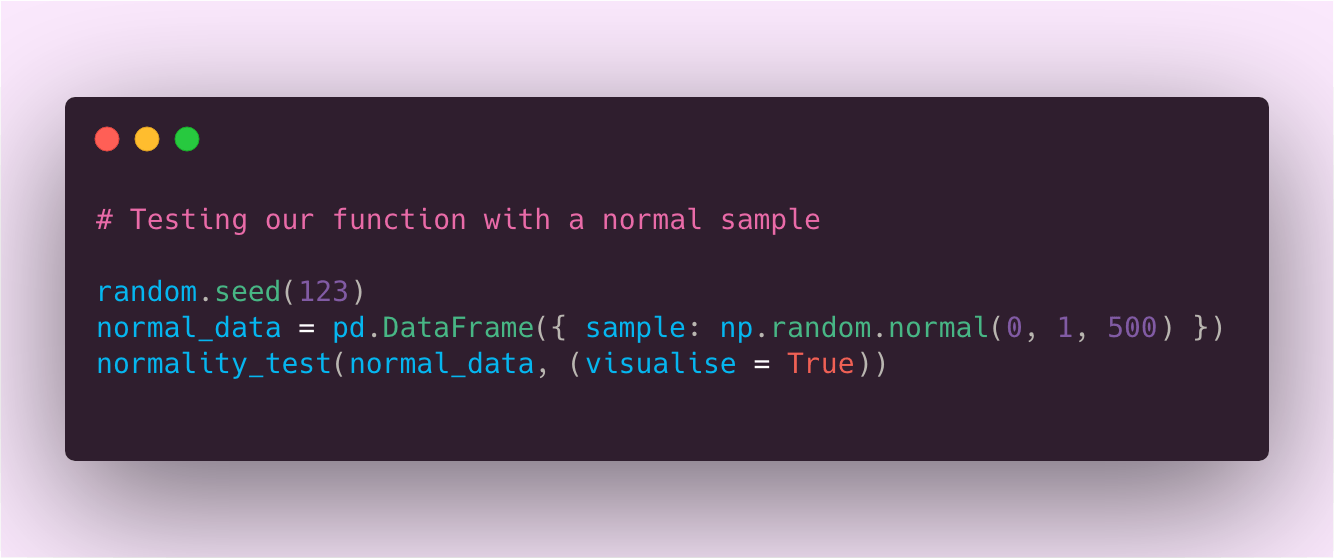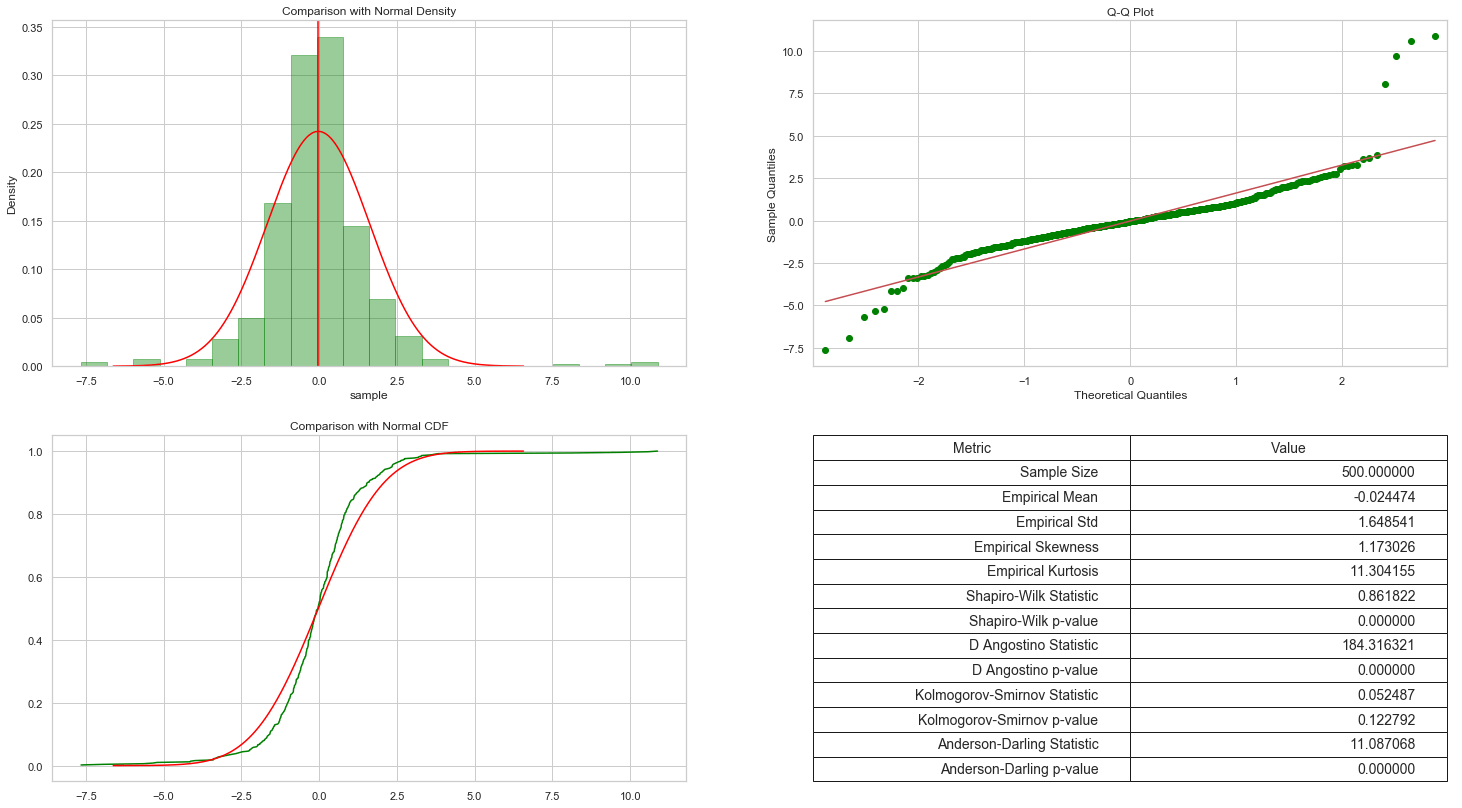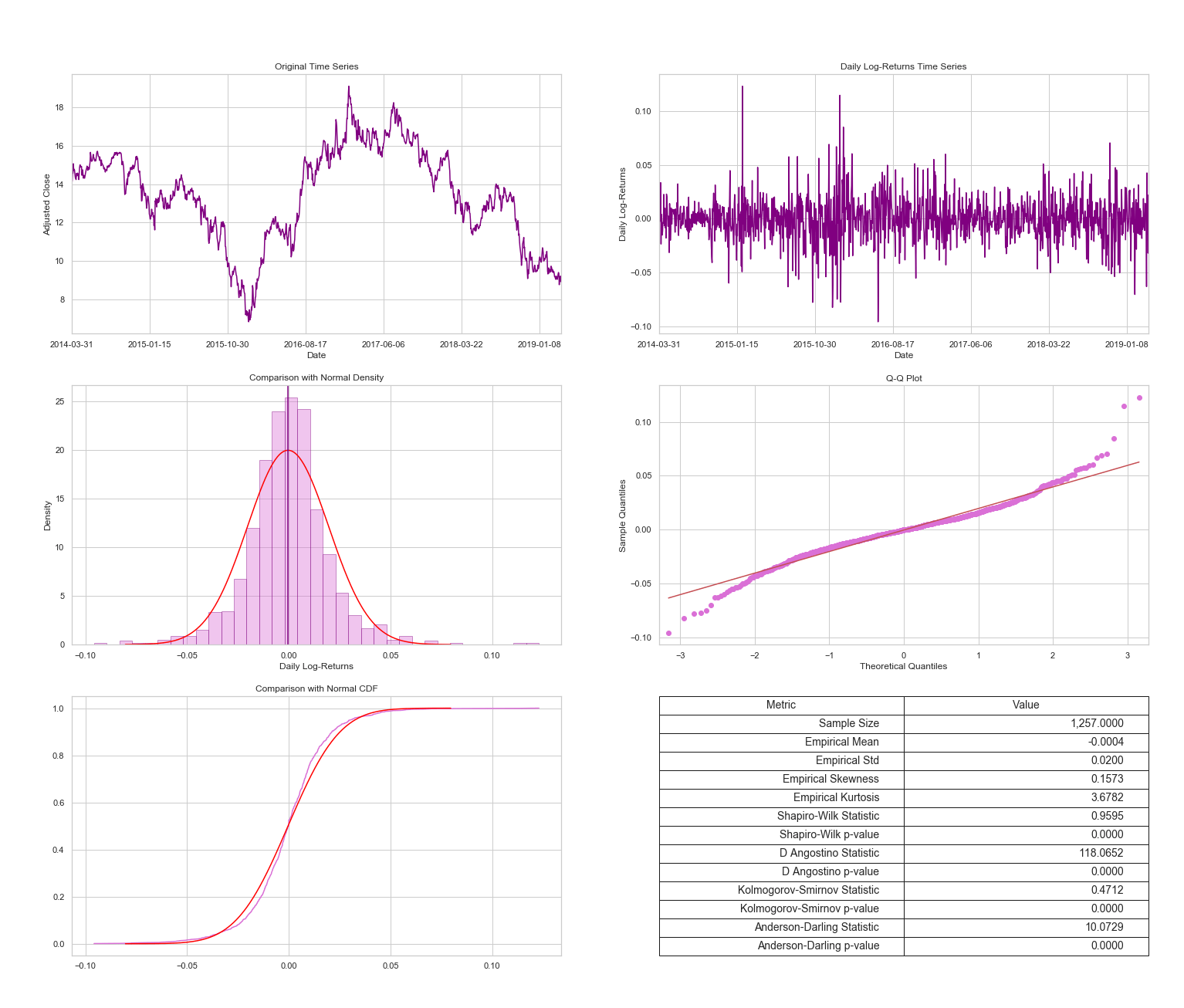# Testing for NormalityA simple Python function to use whenever we are interested in assessing normality. It provides both visual tools and a hypothesis tests.You can find the complete script here. This function provides:

## Visualisations

• Histogram of the returns compared with a normal density plot with parameters equal to the empirical mean and variance
• Quantile – Quantile (Q-Q) plot
• Cumulative Distribution Function (CDF) plot against the normal CDF with parameters given by the empirical mean and variance

## Hypothesis Test

• Shapiro Wilk
• D’ Angostino
• Kolmogorov-Smirnov
• Anderson-Darling

Let’s test the function with some normal data.and now with a sample from a standard t-distribution with 3 degrees of freedom:## Testing Normality: Returns and Log-Returns

It is well-known that many financial models assume that returns or log-returns follow a normal distribution. In these cases, it is a good practice to do some analysis in order to assess departures from normality. Modifying slightly our first function we get a new function which calculates the returns (standard or log-returns) first and then proceeds with the normality assessment.We tested a number of returns for financial time series and none of them passed a single normality test… which is not surprising after looking at the visualisations. You can find more examples in the Python Notebook.  In a future post I will discuss every test more in detail.

This site uses Akismet to reduce spam. Learn how your comment data is processed.